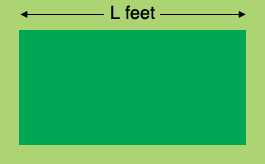SEARCH HOMEMath Central Quandaries & QueriesQuestion from Amber, a student: A rancher wants to use 300ft of fencing to enclose a rectangular area of 4400 square feet. What dimensions should the rectangle be?Hi Amber,

The perimeter of the rectangular enclosure is 300 feet so the length plus the width is 150 feet. I let the length be $L$ feet.What is the width of the enclosure? The length times the width is 4400 square feet. Solve for $L.$

PennyMath Central is supported by the University of Regina and the Imperial Oil Foundation.